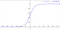Visualizing Support Vector Machine (SVM)

Support Vector Machine is a Supervised machine learning Algorithm used for performing classification as well as regression related tasks. It is a popular choice among the machine learning experts due to its high accuracy and optimization and today we will understand the reason behind it.

Note that the whole blog would be focused only on the geometric intuition of SVM in order to provide a better understanding to the readers.

## Expected Questions to be answered in this blog:

1. What are Support Vectors?

2. How Support Vector Classifier works?

3. What makes it different from Logistic and Linear Regression models?

4. How does…

# Importance Of Sigmoid function in Logistic Regression

Before Understanding the importance of sigmoid function let us understand what Sigmoid function is:

The Sigmoid is a mathematical function with the ‘S-like’ shape. The main objective of the sigmoid function is to scale down or scale up the values within the range of 0 to 1.Graph of Sigmoid function

In neural networks sigmoid is widely used mainly because It is easy to differentiate and since it returns the value within [0,1], it is great for the probabilistic interpretation.

Expression:

# How Logistic Regression classifies?

Topics to be covered in this blog:

1. What is Logistic Regression?
2. How it works over the data points

Logistic Regression is statistical and a supervised learning technique that is widely used in the classification of data with an assumption that it should be almost ‘linearly separable’.

By ‘Linearly Separable’, it means that the output should be binary (Yes/No, True/False). Therefore the objective of the Logistic Regression is to find that PLANE/LINE which separates maximum no of those linearly separable data points.

Now let us proceed towards the mathematical aspect of Logistic Regression. So our objective is to find that line/plane…## Aditya Tiwari

A Machine Learning Junkie